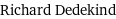# Get Tutoring Near Me!(800) 434-2582

/ Get Math Help

## Dedekind Ring

Alternate name
Definition

A Dedekind ring is a commutative ring in which the following hold. 1. It is a Noetherian ring and a integral domain. 2. It is the set of algebraic integers in its field of fractions. 3. Every nonzero prime ideal is also a maximal ideal. Of course, in any ring, maximal ideals are always prime. The main example of a Dedekind domain is the ring of algebraic integers in a number field, an extension field of the rational numbers. An important consequence of the above axioms is that every ideal can be written uniquely as a product of prime ideals. This compensates for the possible failure of unique factorization of elements into irreducibles.

Related terms
Associated person